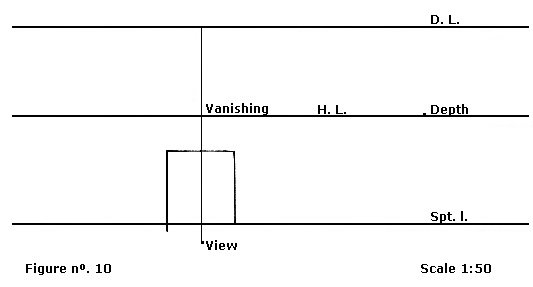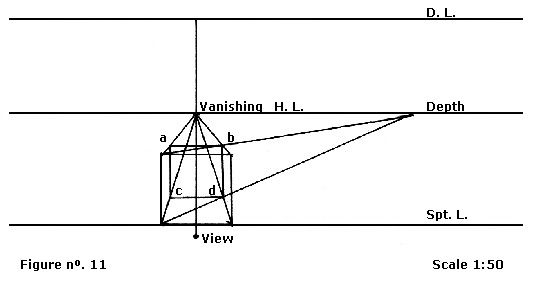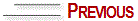# HOW TO DRAW A CUBE IN PARALLEL PERSPECTIVE

Draw to a scale.

A - Locate the three basic lines to find the visual field:-support linehorizon linedistance line.

B - Draw a perfectly geometric square on the support line, with the vertical and horizontal lines parallel between them.

C - Divide the square in the middle and draw at this point a vertical line that goes from the support line to the distance line. Mark the vanishing point at the intersection of this line with the horizon line. Always on the middle of the square and from the support line, mark the visual distance (17cm to scale) and locate the view point.

D - Now find the depth point on the horizon line, placing it far away from the vanishing point, in the same proportion that the view point is located far from the distance line. See figure nº 10.

E - Draw four converging lines to the vanishing point starting from the four vertices of the square on the horizon line, which is the remotest view point.

F - Draw two diagonal lines from the square vertices to the depth point. At the intersections of these lines going to the vanishing point, you will find the cube depths. See figure nº 11. Now, join points a, b, c, d – and you will have a cube constructed to scale in millimeters.© Yvonne Tessuto Tavares - All rights reserved